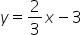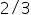Mathematics
Easy

Question

# Write the equation of a line with a slope of 2/3 and a y-intercept of -3.

## y=3/2x+3y=2/3-3y=2/3x-3y=2Hint:

## The correct answer is: y=2/3x-3

### Using the slope and y-intercept of the line to write the equation of the line.Slope-intercept from of equation of a line is y = mx +b[substitutingfor m and -3 for b]

directly substitute values for m and c in the equation.

### Related Questions to study#### With Turito Foundation.#### Get an Expert Advice From Turito.